## Translate

### WHAT IS OTTO CYCLE ? PROCESS EXPLANATION WITH p-V and T-s DIAGRAM.

OTTO CYCLE.It is a gas power cycle that is used in spark ignition engine(i.e. petrol engine) for its working. The entire modern petrol engine works on Otto cycle.This cycle was originally devised by a Frenchman Beau-de-Rochester in 1862. The first successful engine working on this cycle was built by a German engineer Nicholas A. Otto in 1876. These days many gas, petrol and many of the oil engines run on this cycle. It is also known as constant volume cycle as the heat is received and rejected at a constant volume.

This cycle is taken as a standard of comparison for internal combustion engines. For the purposes of comparison with other cycles, the air is assumed to be the working substance. It has low compression ratio ranges from 7:1 to 10:1.The ideal Otto cycle consists of two constant volume and two reversible adiabatic or isentropic processes  as shown on p-v and T-s diagrams in fig.In the above diagrams,
p → Pressure
V → Volume
T → Temperature
s → Entropy
Vc → Clearance Volume
Vs → Stroke Volume

The four processes of this cycle is as follows:

1. Isentropic ( reversible adiabatic) compression
2. Constant volume (Isochoric) heat addition
4. Constant volume heat rejection.

Processes in Otto Cycle:

(To Understand clearly please Refer above p-V and T-s diagrams.)

As stated earlier, Otto cycle consists of four processes. They are as follows:

Process 1-2: Isentropic compression :
In this process, the piston moves from bottom dead centre (BDC) to top dead centre (TDC) position. Air undergoes reversible adiabatic (isentropic) compression. We know that compression is a process in which volume decreases and pressure increases. Hence, in this process, volume of air decreases from V1 to V2 and pressure increases from p1 to p2Temperature increases from T1 to T2As this an isentropic process, entropy remains constant (i.e., s1=s2). Refer p-V and T-s diagrams for better understanding.

Process 2-3: Constant Volume Heat Addition:
Process 2-3 is isochoric (constant volume) heat addition process. Here, piston remains at top dead centre for a moment. Heat is added at constant volume (V2 = V3from an external heat source. Temperature increases from T2 to T3pressure increases from p2 to p3 and entropy increases from s2 to s3
In this process,
Heat Supplied = mCv(T3 – T2)
where,
m → Mass
Cv → Specific heat at constant volume

Process 3-4: Isentropic expansion :
In this process, air undergoes isentropic (reversible adiabatic) expansion. The piston is pushed from top dead centre (TDC) to bottom dead centre (BDC) position. Here, pressure decreases for p3 to p4volume rises from v3 to v4temperature falls from T3 to T4 and entropy remains constant (s3=s4).

Process 4-1: Constant Volume Heat Rejection :
The piston rests at BDC for a moment and heat is rejected at constant volume (V4=V1). In this process, pressure falls from p4 to p1temperature decreases from T4 to T1 and entropy falls from s4 to s1
In process 4-1,
Heat Rejected = mCv(T4 – T1)

The efficiency of the Otto cycle also known as ideal efficiency or air standard efficiency is given by:

The starting point is the general expression for the thermal efficiency of a cycle: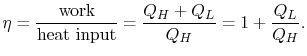The convention, as previously, is that heat exchange is positive if heat is flowing into the system or engine, so QL is negative. The heat absorbed occurs during combustion when the spark occurs, roughly at constant volume. The heat absorbed can be related to the temperature change from state 2 to state 3 as: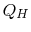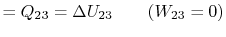The heat rejected is given by (for a perfect gas with constant specific heats)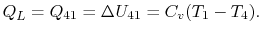Substituting the expressions for the heat absorbed and rejected in the expression for thermal efficiency yieldsWe can simplify the above expression using the fact that the processes from 1 to 2 and from 3 to 4 are isentropic: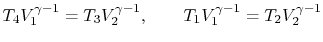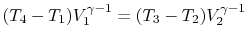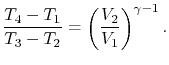The quantity V1/V2=r  is called the compression ratio. In terms of compression ratio, the efficiency of an ideal Otto cycle is: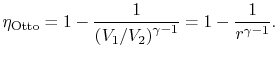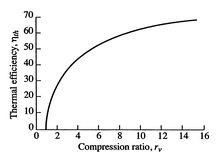Ideal Otto cycle thermal efficiency
The ideal Otto cycle efficiency is shown as a function of the compression ratio in above  Figure . As the compression ratio,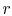, increases,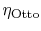increases, but so does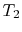. Ifis too high, the mixture will ignite without a spark (at the wrong location in the cycle).

NOTES :-
1. We see from equation {4} that the efficiency of the Otto cycle depend on compression ratio (r).
2. The efficiency increases with compression ratio (r). In actual practice, r can not be increased beyond a value of 7 or so.
3. Compression ratio,
r = Total cylinder volume/ Clearance volume = Clearance volume + Stroke volume/ Clearance volume.
Clearance volume = Stroke volume/ r - 1.